# Totals (SUM)

Contents[Hide]

The Sum function calculates the total sum for any number of input value series.

## 1. Syntax

```SUM(d0,Alignments...)
```

## 2. Input

The Sum function requires the following input:

• d0 - The set of data values for which the Sum is calculated.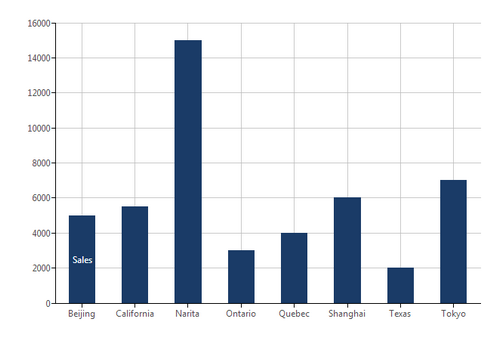Formula - Sum: Input data

## 3. Parameters

The Sum function requires the following parameters:

• Alignments... (One or More, Optional) – Hierarchy placeholders to be used as the alignment axis.

### 3.1. Add the formula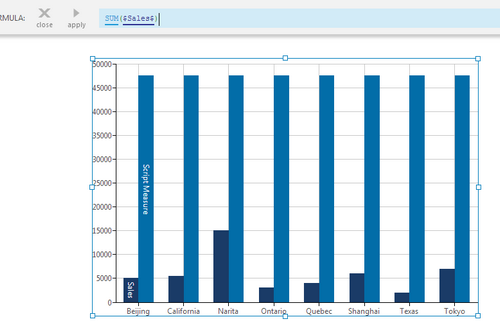Add the formula

## 4. Output

The Sum function generates the following output:

• Total - A single value representing the total sum of the input values.

### 4.1. Change the chart type (optional)

For example, changing the chart type of the series derived from the formula from a Bar Chart to a Line Chart.

1. Click the series, right click, and select Re-visualize.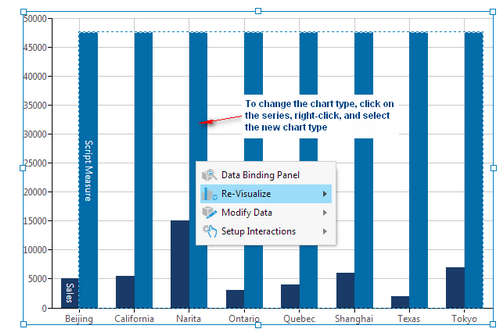Re-visualize

2. Select the new chart type.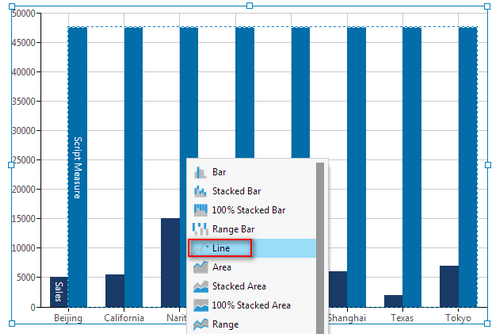Choose the Chart type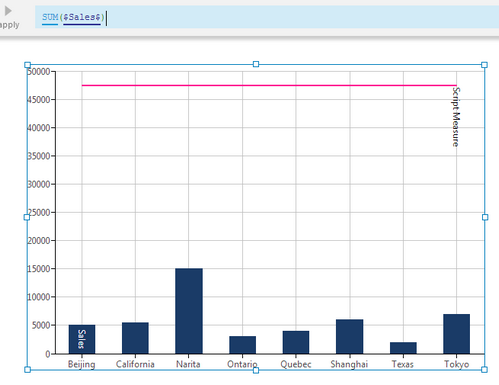Sum: Output

To display the output in a separate control, such as a Data Label, you can use a formula visualization.

## 5. See also

Dundas Data Visualization, Inc.
500-250 Ferrand Drive
Toronto, ON, Canada
M3C 3G8

North America: 1.800.463.1492
International: 1.416.467.5100

Dundas Support Hours: 7am-6pm, ET, Mon-Fri